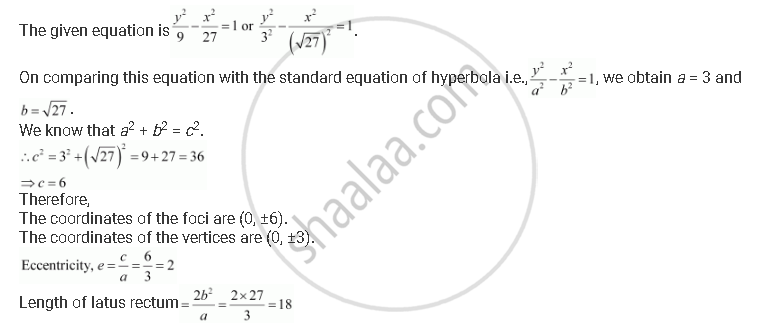CBSE (Arts) Class 11CBSE
Share
Notifications

View all notifications
Books Shortlist
Your shortlist is empty

# Find the Coordinates of the Foci and the Vertices, the Eccentricity, and the Length of the Latus Rectum of the Hyperbola Y^2/9 - X^2/27 = 1 - CBSE (Arts) Class 11 - Mathematics

Login
Create free account

Forgot password?
ConceptHyperbola Eccentricity

#### Question

Find the coordinates of the foci and the vertices, the eccentricity, and the length of the latus rectum of the hyperbola y^2/9 - x^2/27 = 1

#### SolutionIs there an error in this question or solution?

#### APPEARS IN

Solution Find the Coordinates of the Foci and the Vertices, the Eccentricity, and the Length of the Latus Rectum of the Hyperbola Y^2/9 - X^2/27 = 1 Concept: Hyperbola - Eccentricity.
S